## Pages

### Fun Pythagorean Theorem Activities and Teaching Ideas

This is a bit embarrassing, but because I try my best to swallow my pride and embrace growth mindset, here I am fessing up... It wasn't until my first year teaching that I finally realized what a2 + b2 = c2 really meant. The teacher next to me had done a Pythagorean Theorem activity and decorated the halls with his students' finished work. It was looking at all of those projects that finally drove home that the theorem had a real, concrete proof. It was a cool realization. It was this same teacher who made me realize the value of math word walls and displaying student work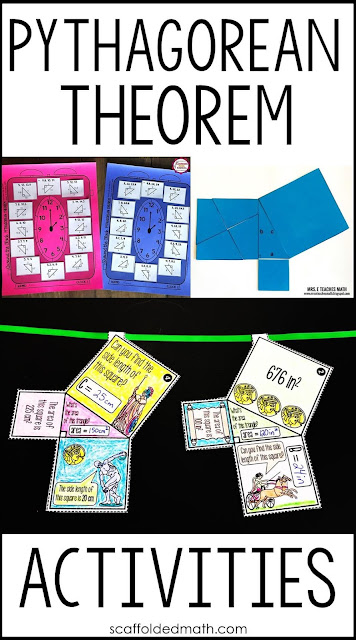Since that time, I have gone all in on math word walls because of how well they work for my students. And more recently, I converted all of my math word walls to include digital versions so that students working online can still access to their vocabulary references. I added a Pythagorean Theorem reference to this digital math word wall for Geometry:digital geometry word wall - home

Clicking on the Pythagorean Theorem image from the home screen above opens up a room where the Pythagorean Theorem, Distance and Midpoint formulas are all displayed:Pythagorean Theorem room

To be fair to myself about the whole Pythagorean Theorem proof situation from above, I had started as a biology teacher teaching algebra and hadn't seen the inside of a geometry book since I myself had been in high school. But I'll never forget those Geometry projects. They were a nice reminder that, however old we are, we never stop learning.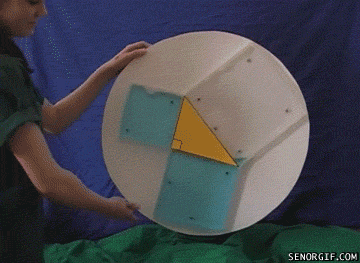Pythagorean Theorem water proof

If only there had been GIFs when I was in high school!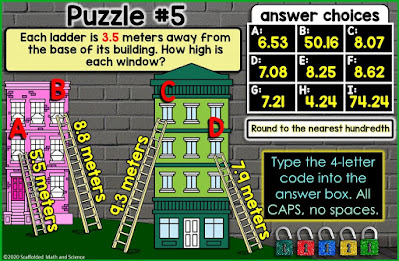Pythagorean Theorem digital math escape room - puzzle #5

Converting all of those math word walls to digital finally ended my digital foot-dragging and I am now thoroughly enjoying all of the possibilities that come along with making digital activities. Self-checking, color, no paper or ink. The color is probably my favorite thing. I've been in such a mindset to only create things that can be printed in black and white that all the color options have been a whole new world. Here are puzzles 5 and 3 of a Pythagorean Theorem digital math escape room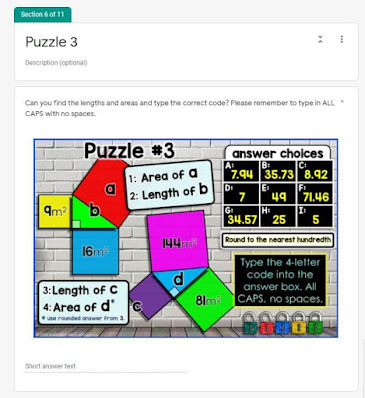Pythagorean Theorem digital math escape room - puzzle #3

This escape room covers finding missing leg and hypotenuse lengths, plus some area questions to bring in prior knowledge. The digital math escape rooms I've been making are answer-validated Google Forms with no outside links. Everything is right there in the Form. Students enter codes to unlock each puzzle lock. I wanted to make them easy for teachers to use and fun for students.

Back in 2004 Teachergram wasn't yet a thing. If it was, I'm sure things would have been very different about my first years of teaching. These days with social media, the ideas are constantly flying, making us all better teachers. And learners!

Through social media, blogging and TpT, I've also made some good math teacher friends (who I lovingly refer to as my "math friends") and in this post I want to share some of their fun Pythagorean Theorem activities.If proving the Pythagorean Theorem had been a thing when I was in 8th grade math, maybe my first year of teaching wouldn't have been so... eye-opening. Amanda from Free to Discover made this Pythagorean Theorem Proof Discovery Worksheet to prove the theorem.Kara from Learning Made Radical has been making these great math bookmark notes that I can just imagine kids referring to late at night while doing homework. She made this set of Pythagorean Theorem Bookmark Notes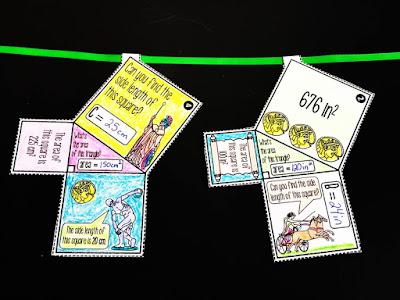Pythagorean Theorem math pennant activity

It's hard for me to believe that I've made over 100 math pennantsOf all of them, this Pythagorean Theorem Math Pennant was one of the most difficult to make because of all of the graphics. Students find missing side lengths of the inner right triangles, areas and perimeters.Kara from Learning Made Radial and I have been collaborating on partner scavenger hunt activities that get students working together on their math.

I had a senior a few years ago who had a boyfriend who she never spoke to face-to-face. This got me thinking about kids and how they need time during their day for meaningful conversation. Kara made this Pythagorean Theorem Partner Scavenger Hunt activity.Kara also made this fun Pythagorean Theorem Hole Punch GameKarrie from Mrs. E Teaches Math made this Pythagorean Theorem Word Problem Coloring Sheet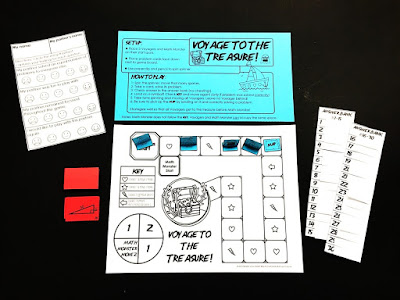Pythagorean Theorem Voyage to the Treasure! game

Alex from Middle School Math Man and I have been collaborating on a new math game series called Voyage to the Treasure!Pythagorean Theorem Voyage to the Treasure! game

In these new math games, students are on the same team, working through math problems as they try to beat the Math Monster to the treasure. The one above is Voyage to the Treasure! Pythagorean Theorem.Mel from Secondary Math Shop made this Pythagorean Theorem Cut, Paste, Solve and Match puzzle where students set up and solve Pythagorean Theorem problems.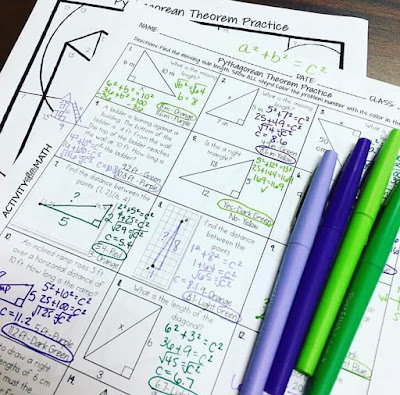Hayley from Activity After Math made this Pythagorean Theorem Color By Number activity where students find a missing leg, hypotenuse, converse or the distance between two points.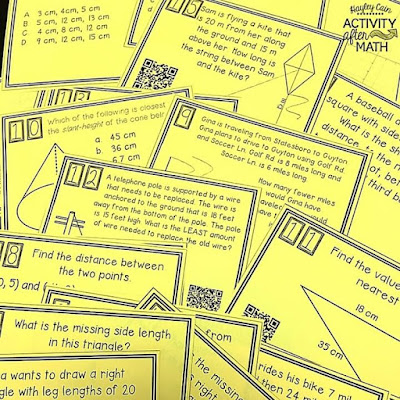Hayley also made this set of Pythagorean Theorem Task Cards with QR codes.

I've always had a lot of success with scavenger hunts with my students. They really seem to enjoy them. Alex made this Pythagorean Theorem Scavenger Hunt for 8th grade math students.Karrie wrote a great post called The Pythagorean Theorem Without Words over on her blog. The image above from her post is Perigal's Puzzle.

Karrie also pulled together a list of her favorite Pythagorean Theorem resources in her post 7 Ideas for Teaching the Pythagorean Theorem.

If you have Geometry students who speak Spanish, this wordless Pythagorean Theorem proof has some funny outtakes at the end! Actually, they're even funny if you don't speak Spanish.

I hope you are having a wonderful year!